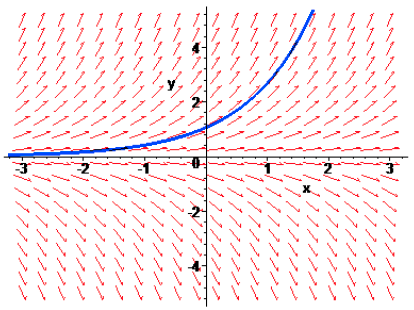## A Natural Question

### The Question

Recall that the natural log function $\ln x$ has an understood base of $e \approx 2.71828$. What is so "natural" about base $e$?

Nicholas Mercator was the first to coin the term “natural log” (actually, he used the Latin equivalent log naturalis), in his treatis Logarithmo-technica on logarithms, published in 1668. He noticed that there are a number of simple series involving the natural logarithm. For instance,

$$\ln(x+1) = x - \frac{1}{2} x^2 + \frac{1}{3} x^3 - \frac{1}{4} x^4 + \cdots$$

The most natural properties of e, however, are found in its relation to calculus— which, strangely, wasn’t even developed until after Mercator’s work.

Recall that much of mathematics, as we practice it today, involves changing one thing into something else. Arithmetic operations like addition and subtraction change two numbers into a sum or a difference. The functions encountered in basic algebra generally change one real number into another one by means of some explicit formula. Differentiation and integration, as explored in Calculus, give us a way to change functions into other functions. The list continues. As such, a central and fundamental question in mathematics is this: Given some process for changing one thing into another, when does this process fail to create a change?

For example, recall that the differentiation operator, $\frac{d}{dx}$, takes an initial function $f(x)$ and changes it into another function (called the derivative of $f(x)$) that gives the slope of the tangent line to the curve of $y=f(x)$ at any value $x$. Specificall, this derivative function is found by determining the following limit:

$$\frac{d}{dx} f(x) = \lim_{h \rightarrow 0} \frac{f(x+h) - f(x)}{h}$$

So when does this process fail to create a change—that is to say, when does the operation of differentiation result in the same function with which one started? Thus, we are looking for a function that satisfies

$$\frac{d}{dx} f(x) = f(x)$$

To get an idea about what such a function might look like, let us draw an arrow with slope y at several points on the coordinate plane, so that we can attempt to draw a function that appears to follow these slopes.It should not be too difficult to see the nature of the functions that satisfy $\frac{d}{dx} f(x) = f(x)$. Notice their apparent unbounded growth (or decline) on the right and a horizontal asymptote on the left. One might make a guess that the functions in question are exponential—and one would be right!

Working from this assumption, let us assume some simple exponential function of the form $f(x) = b^x$, when differentiated, equals itself. Now the questions becomes: "What value(s) of $b$ will produce such a function?" Starting with the limit definition of the derivative, we then have

$$\lim_{h \rightarrow 0} \frac{f(x+h) - f(x)}{h} = f(x)$$

which, for this particular $f(x)$, requires

$$\lim_{h \rightarrow 0} \frac{b^{x+h} - b^x}{h} = b^x$$

Equivalently, for sufficiently small $h$,

$$\frac{b^{x+h} - b^x}{h} \approx b^x$$ $$\frac{b^x(b^h - 1)}{h} \approx b^x$$ $$\frac{b^h - 1}{h} \approx 1$$

At this point, we can "solve" for $b$ to find that, for sufficiently small $h$,

$$b \approx (1+h)^{1/h}$$

To make this a little easier to calculate, one might consider replacing $h$ with $1/n$, since taking $n$ sufficiently large, we can make $1/n$ as small as we like. With this change, we find that for sufficiently large $n$,

$$b \approx \left(1 + \frac{1}{n} \right)^n$$ Of course, as you have probably guessed, $$\lim_{n \rightarrow \infty} \left(1 + \frac{1}{n} \right)^n = 2.71828\ldots$$

So an exponential function that has a base of e behaves in the nicest possible manner under differentiation (and integration for that matter).1 As such, e is the “natural” choice for the base of an exponential function. Finally, since log functions are the inverses of exponential functions with the same base, e is the natural choice there as well.

### Implications for the Derivatives of $e^x$ and $\ln x$

Knowing from the above discussion that

$$\frac{d}{dx} e^x = e^x,$$

we can also now quickly find the derivative of the natural logarithm function, $\ln x$.

Suppose $y = \ln x$. Then

$$e^y = x$$

Differentiating this equation implicitly with respect to $x$, we have

$$e^y \frac{dy}{dx} = 1$$

Dividing both sides by $e^y$, we have a formula for $dy/dx$ -- although granted it is in terms of $y$ and not $x$, which would be more desirable.

$$\frac{dy}{dx} = \frac{1}{e^y}$$

However, that difficulty is quickly overcome, since we know $e^y = x$. Upon making this substitution, we have

$$\frac{dy}{dx} = \frac{1}{x}$$

Equivalently,

$$\frac{d}{dx} \ln x = \frac{1}{x}$$

1It should be noted, that any constant multiple of $e^x$ is also its own derivative, and this accounts for all of the other functions that one could draw in agreement with the previously shown slopefield.